Next: mW Results Up: W Mass by Direct Previous: Example of Calorimeter Uncertainties

## Using Z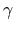Events

The emission of a hard ISRreduces the effective centre-of-mass energy of e+e- interactions, such that the effective interaction can be at the Z resonance. Kinematic reconstruction of these so-called Z'' events, with Z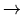q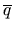, can provide a cross-check to W mass measurements, by measuring either mZ or Ebeam, and comparing these values to independent measurements.

L3 measure mZ from qevents  at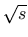= 189 GeV using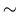10K events. This is a check of detector calibration, jet reconstruction, fitting method etc., and uses a kinematic fit and a reweighting technique as in their W mass analysis. The fitted value from Zevents is mZ = 91.106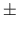0.062 GeV (prel.), in good agreement with mZ extracted from their cross-section measurements at the Z-pole of mZ = 91.1950.009 GeV. This represents an important test of the complete mass analysis method.

Since the beam energy is used in the kinematic fits of WW events, the uncertainty on it propagates through to the W mass measurement. The error on the beam energy is very precise at LEP1, less than 1 MeV, as it is measured by resonant depolarisation. Polarisation has not been achieved above a beam energy of 60 GeV, so the same technique does not work at LEP2. Instead, beam energy measurements at lower beam energies are extrapolated to higher energies, resulting in a larger beam energy error (25 MeV at= 183 GeV ). As a cross-check of the LEP determination of ECM, ALEPH use the qevents by providing the precise ( mZ,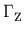) information and using the jet angles to extract ECM. The average LEP centre-of-mass energy at ALEPH for the= 183 GeV run of 1997 is measured to be 182.500.19(stat)0.08(syst) GeV , which is consistent with the estimate from the LEP energy working group  of 182.6520.050 GeV.Next: mW Results Up: W Mass by Direct Previous: Example of Calorimeter Uncertainties
latex2html conversion by www person on 1999-12-05